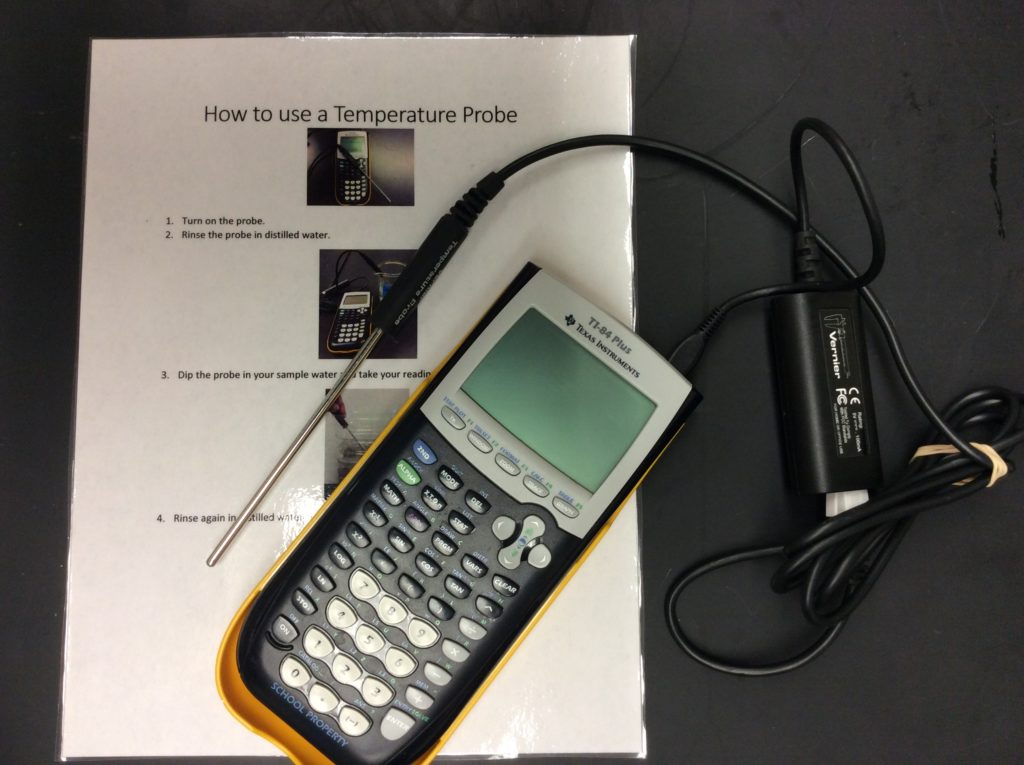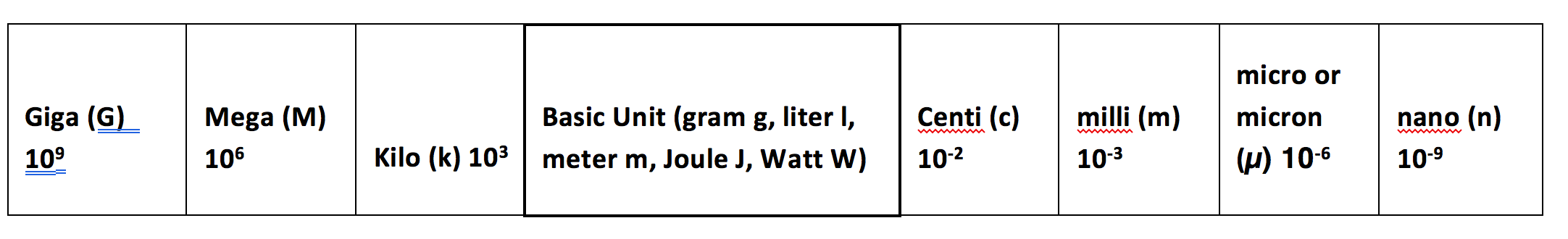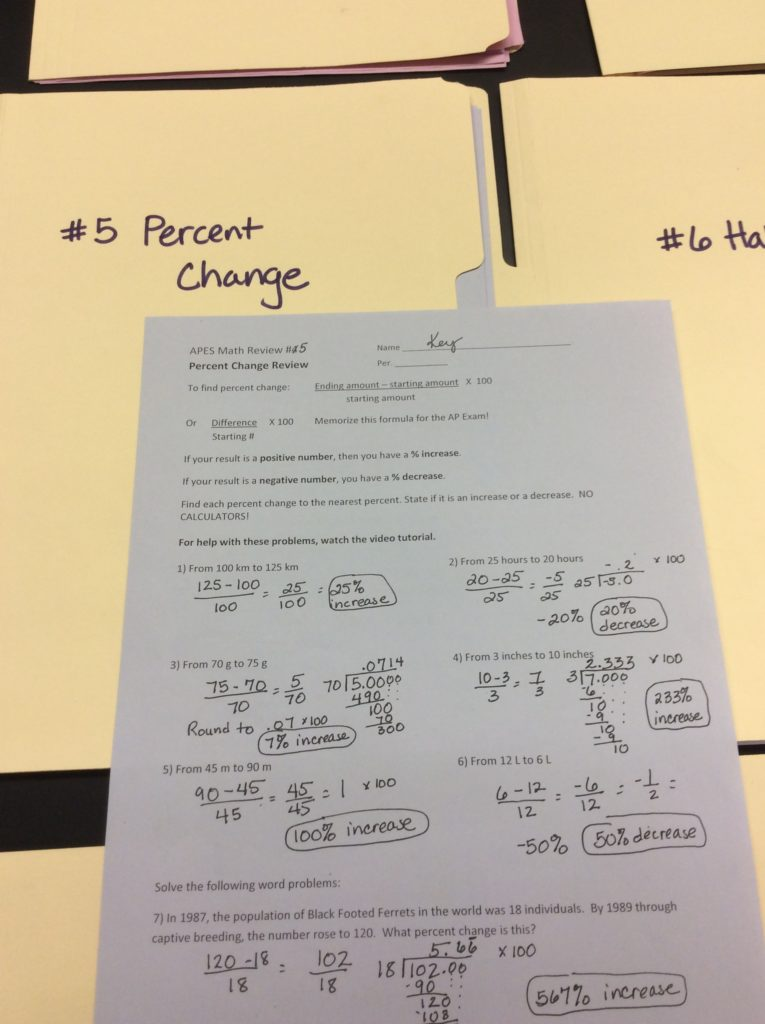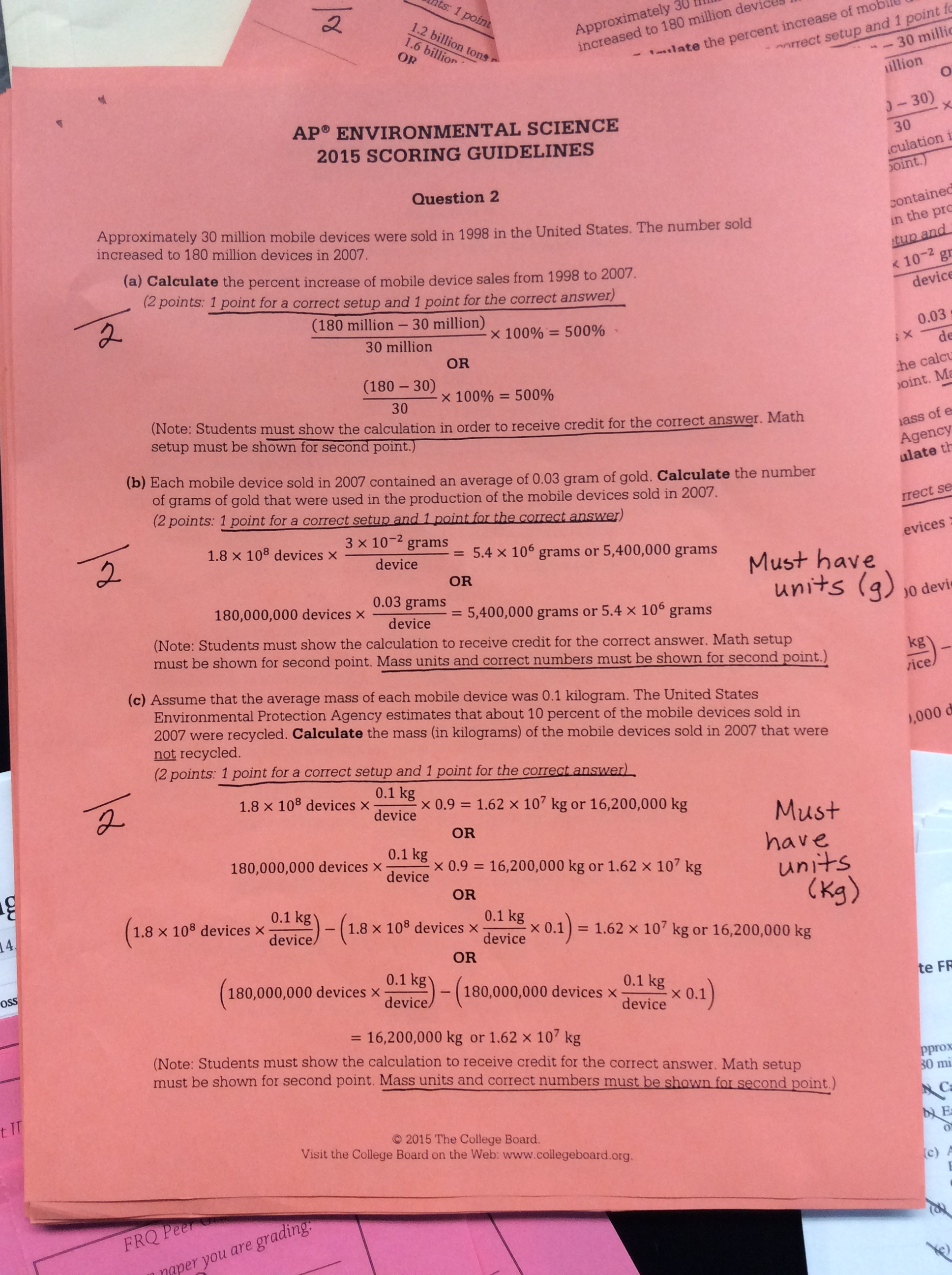# APES Math

There are many ways to practice AP® Environmental Science (APES) math.  As a teacher, you will decide which approach is best your own students.  You may have students who find the math super easy and some that find it impossible. APES math practice problems can be found from many sources–textbooks, shared drives, and released FRQs.

# APES Math Info

This is the basic information you need about APES math on the AP® Exam. (Updated July 2019 to reflect the new APES Calculator policy and the APES exam changes)

1. Calculators:  Students may use calculators now. Refer to the AP® calculator policy on the College Board Website.This is a no-no for the AP® Exam, but useful for a temperature probe!
2. Pre-Algebraic Word Problems. Many students don’t know how to apply math to life problems.  Even kids who are in higher level math (like pre-calculus) still struggle with these word problems.  The biggest hurdle is often setting up the problem.
3. 6-9% on the Multiple Choice Section. There are 80 MC questions total and 4-8 are math.  The Rule of 70 has been a favorite for on the MC for many years. Kids DO NOT need to show work for MC questions.
4. One FRQ is Half Math. There are 3 mandatory FRQs in 80minutes. Question #3 will have math for half the problems. Over the years, the math has gotten easier on the FRQs, but this has not increased the national pass rate.
5. Students MUST Show Work on FRQs. NO WORK=NO CREDIT, even if correct. Units in set-up and in answer.
6. No Formula Sheet. Students must memorize some simple formulas (see below).

A student can pass the AP® Exam without doing any of the math, BUT this is a gamble and they have to know everything else really, really well.

When I analyze my AP® results, the math FRQ performs the weakest and often drops kids from a 3 to a 2. This is why I focus on math a lot.

# Math Skills Kids Should Have Already Learned (But Probably Forgot)

1. Dimensional Analysis. This is something learned in chemistry, but often forgotten. APES math always has dimensional analysis.
2. Density: Also in chemistry… and in middle school …and maybe elementary school.
3. pH: Just the basics.
4. Half-Life: Often taught in chemistry and/or physics. This may be new for freshmen in APES.
5. Scientific Notation. A favorite on the AP® Exam and one that most kids really don’t like. Many students will convert to zeros before solving, but this is a gamble as more errors are made when they do that. The AP® Exam will often use scientific notation in the givens and these divide or multiply cleanly so its a benefit to know this skill.
6. Long Division by hand. Many students have forgotten this elementary math skill and struggle with it.
7. Percentages.  Many students don’t remember how add, subtract, multiply or divide using percentages without a calculator.
8. Percent Change
9. Metric Conversions

# New Math in APES (for most kids)

1. Population Math (see below)
2. Productivity (see below)
3. Trophic Levels (90% loss of energy as you go up a trophic level)
4. Energy Math: Kilowatts, joules etc.
5. LD-50 calculations

# Formulas they need to memorize

1. Population Math Basic, easy formulas
2. Percent Change3. Metric Conversions–g to kg, for example. They need to know the following prefixes only: micro, milli, centi, kilo, and Mega.The table from my metric review.
4. Density–one they should know, but need to be reminded of
5. pH scale1 # decrease on scale = 10x H+ (acidity)
6. Productivity:  Gross Primary Production – Respiration = Net Primary Productivity
7. Half-Life–not a formula, but a method to solve by sketching outSample half-life problem with solution

Students DO NOT need to memorize any other conversions such as gallons to liters.

# Methods to Tackle the Math

There are many ways to tackle APES math review and to teach new skills. You have to try different methods to figure out what works and what doesn’t work with your particular students.

I have a mixed class with 1/3 of the kids who find the math easy, 1/3 who need some review and they’re fine, and 1/3 who find APES math extremely challenging. I’ve tried many different methods over the years.

Here are some various ways to tackle the math that I’ve tried or other teachers have tried.

1. Math diagnostic. A math diagnostic is helpful to see where your students are weak as a class or as an individual if you have mixed abilities. Then, students can do the review papers they need instead of all of the review. During math review, you can differentiate the approach students take by giving them choices.
2. Math as a summer assignment. I don’t do this, but some teachers find this effective.
3. Review papers.  Can be done in class or for homework.  I prefer in-class to prevent copying and so I can differentiate. I flipped to allow more time to do this.  My students work on the review they need and have several options for how to do it.  They can watch a video for help (see below) for the whole paper, they can do the problems on their own and then check with a key. Or, they can do a combination of the two.Review papers with keys.
4. Practice Problems for new math.  Some kids will find new math (population, energy math etc) easy and some find it difficult. I sometimes differentiate by allowing them some choices in learning and practicing. I have videos made of all my worksheets (see below) . Here’s one way I introduced the choices to the kids.
5. Add math to labs wherever you can. Make it dimensional analysis.Owl Pellet Lab with dimensional analysis questions
6. Add math problems to Multiple Choice Tests. Here are some I recently made for a toxicology and cities exam. (Chapters 13 and 14 Withgott).
7. FRQ math practice.  Use released FRQs to do this.  We often peer-grade these FRQs so the kids can really understand how the CB grades them at the reading.Scoring guidelines for FRQ #2. Students use this paper to peer-grade.
8. Review videos. I have recorded solutions to all my math papers so that students can watch me solve if they get stuck.#### Kristi Schertz

View all posts by Kristi Schertz →